DIY press

Under socialism regime was in some socialist countries to own a typewriter requires special permission. That has hindered the spread of DIY literature (manually transcribed through carbon copy paper for typewriters).

Calculate how many typewriters today can indulge Joseph with payment 934 euros if typewriter weighs 10 kg and scrap iron is worth 0.19 euro/kg.

Result

n =  492

Solution:Leave us a comment of this math problem and its solution (i.e. if it is still somewhat unclear...):Be the first to comment!To solve this verbal math problem are needed these knowledge from mathematics:

Need help calculate sum, simplify or multiply fractions? Try our fraction calculator.

Next similar math problems:

1. Mixed2improperWrite the mixed number as an improper fraction. 166 2/3
2. Train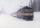The train passes part of the line for 95 minutes at speed 75 km/h. What speed would have to go in order to shorten the driving time of 20 minutes?
3. Classroom 4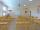In a class of 36 pupils, 2/3 are girls. How much it is in a class girls and boys?
4. Fraction and a decimalWrite as a fraction and a decimal. One and two plus three and five hundredths
5. Scouts 4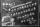4/7 of the students in a school are boys. If 3/8 of the boys are scouts, how many scouts are there in a school of 1878 students?
6. Icecreams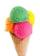Karthik bought 30 icecreams for his birthday .2/5 of them are chocolate icecreams. Find the number of chocolate icecreams he bought?
7. 3 children3 children had to divide 4 pounds is candy. How much candy did each child get?
8. Lengths of the poolMiguel swam 6 lengths of the pool. Mat swam 3 times as far as Miguel. Lionel swam 1/3 as far as Miguel. How many lengths did mat swim?
9. Ratio 11Simplify this ratio 10 : 1/4
10. Fractions 3Calculate 1/9 of 27:
11. In fractionsAn ant climbs 2/5 of the pole on the first hour and climbs 1/4 of the pole on the next hour. What part of the pole does the ant climb in two hours?
12. ZdeněkZdeněk picked up 15 l of water from a 100-liter full-water barrel. Write a fraction of what part of Zdeněk's water he picked.
13. CakesOn the bowl were a few cakes. Jane ate one-third of them, Dana ate a quarter of those cakes that remained. a) What part (of the original number of cakes) Dana ate? b) At least how many cakes could be (initially) on thebowl?
14. The tap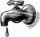For one day flows 148 l of water out of the tap. How much water will flow out for 3/4 day?
15. Seeds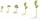Of the 72 wet seeds 7/8 germinated. How many seeds germinate?
16. Write 3Write a real world problem involving the multiplication of a fraction and a whole number with a product that is between 8 and 10 then solve the problem
17. Pie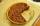Vili ate three pieces of pie . If it piece is 1/8 how much pie did he eat?